# SISO

Single Input Single Output continuous control block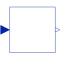# Information

This information is part of the Modelica Standard Library maintained by the Modelica Association.

Block has one continuous Real input and one continuous Real output signal.

# Connectors (2)

u y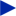Type: RealInput Description: Connector of Real input signal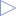Type: RealOutput Description: Connector of Real output signal

# Extended by (43)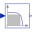Filter Modelica.Electrical.PowerConverters.ACDC.Control PT1 + all-pass filter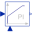LimitedPI Modelica.Electrical.Machines.Examples.ControlledDCDrives.Utilities Limited PI-controller with anti-windup and feed-forward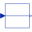RealPassThrough Modelica.Blocks.Routing Pass a Real signal through without modification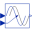VariableDelay Modelica.Blocks.Nonlinear Delay block with variable DelayTime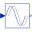PadeDelay Modelica.Blocks.Nonlinear Pade approximation of delay block with fixed delayTime (use balance=true; this is not the default to be backwards compatible)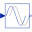FixedDelay Modelica.Blocks.Nonlinear Delay block with fixed DelayTime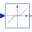DeadZone Modelica.Blocks.Nonlinear Provide a region of zero output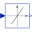SlewRateLimiter Modelica.Blocks.Nonlinear Limits the slew rate of a signal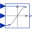VariableLimiter Modelica.Blocks.Nonlinear Limit the range of a signal with variable limits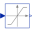Limiter Modelica.Blocks.Nonlinear Limit the range of a signal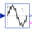TotalHarmonicDistortion Modelica.Blocks.Math Output the total harmonic distortion (THD)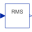RootMeanSquare Modelica.Blocks.Math Calculate root mean square over period 1/f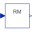RectifiedMean Modelica.Blocks.Math Calculate rectified mean over period 1/f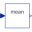Mean Modelica.Blocks.Math Calculate mean over period 1/f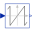WrapAngle Modelica.Blocks.Math Wrap angle to interval ]-pi,pi] or [0,2*pi[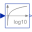Log10 Modelica.Blocks.Math Output the base 10 logarithm of the input (input > 0 required)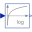Log Modelica.Blocks.Math Output the logarithm (default base e) of the input (input > 0 required)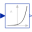Power Modelica.Blocks.Math Output the power to a base of the input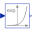Exp Modelica.Blocks.Math Output the exponential (base e) of the input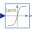Tanh Modelica.Blocks.Math Output the hyperbolic tangent of the input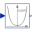Cosh Modelica.Blocks.Math Output the hyperbolic cosine of the input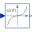Sinh Modelica.Blocks.Math Output the hyperbolic sine of the input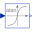Atan Modelica.Blocks.Math Output the arc tangent of the input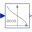Acos Modelica.Blocks.Math Output the arc cosine of the input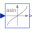Asin Modelica.Blocks.Math Output the arc sine of the input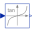Tan Modelica.Blocks.Math Output the tangent of the input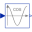Cos Modelica.Blocks.Math Output the cosine of the input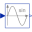Sin Modelica.Blocks.Math Output the sine of the input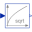Sqrt Modelica.Blocks.Math Output the square root of the input (input >= 0 required)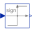Sign Modelica.Blocks.Math Output the sign of the input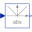Abs Modelica.Blocks.Math Output the absolute value of the input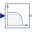Filter Modelica.Blocks.Continuous Continuous low pass, high pass, band pass or band stop IIR-filter of type CriticalDamping, Bessel, Butterworth or ChebyshevI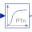CriticalDamping Modelica.Blocks.Continuous Output the input signal filtered with an n-th order filter with critical damping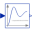LowpassButterworth Modelica.Blocks.Continuous Output the input signal filtered with a low pass Butterworth filter of any order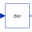Der Modelica.Blocks.Continuous Derivative of input (= analytic differentiations)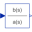TransferFunction Modelica.Blocks.Continuous Linear transfer function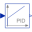PID Modelica.Blocks.Continuous PID-controller in additive description form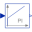PI Modelica.Blocks.Continuous Proportional-Integral controller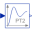SecondOrder Modelica.Blocks.Continuous Second order transfer function block (= 2 poles)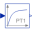FirstOrder Modelica.Blocks.Continuous First order transfer function block (= 1 pole)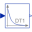Derivative Modelica.Blocks.Continuous Approximated derivative block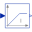LimIntegrator Modelica.Blocks.Continuous Integrator with limited value of the output and optional reset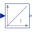Integrator Modelica.Blocks.Continuous Output the integral of the input signal with optional reset